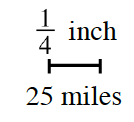### Home > MC1 > Chapter 6 > Lesson 6.2.5 > Problem6-97

6-97.

James lives in Columbus, OH, and wants to know how far he is from Indianapolis. On his map, the two cities are $1\frac{3}{4}$ inches apart. At the bottom of the map, he sees the scale at right. How far apart are Columbus and Indianapolis? How do you know?If one-fourth of an inch is equal to $25$ miles, you will need to find how many one-fourths are in one and three-fourths. Can you first find how many one-fourths are in $1$?

James drew this diagram to help find how many one-fourths are in $1$. Can you complete the diagram to find how many one-fourths are in three-fourths?The diagrams show that there are $7$ one-fourths in one and three-fourths. Use multiplication and the scale above to find how many miles there are from Columbus to Indianapolis.

James lives $175$ miles from Indianapolis. Did you remember to explain how you know?